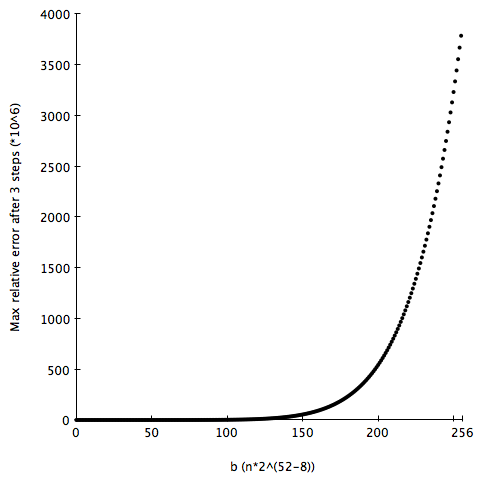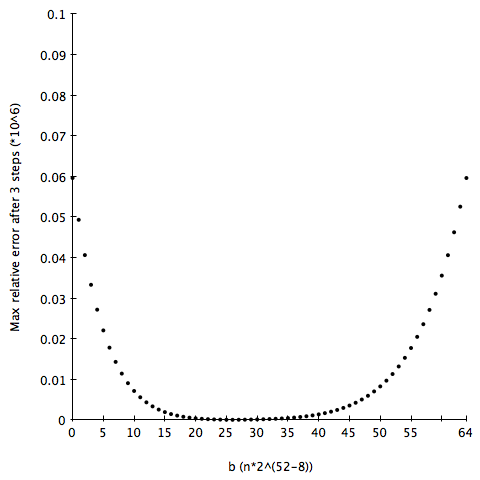# Paul Khuong mostly on Lisp

## Wed, 16 Mar 2011

### 0x7FDE623822FC16E6 : a magic constant for double float reciprocal

EDIT: I never expected this post to garner so much interest. Just to be clear, using the constant is a very bad idea in almost every situation I can think of. Even instructions like `RCPSS `should only be used by people who are convinced that the tradeoffs are worth it (or don’t have any other choice). Subtracting from the constant above is even worse, and handles even fewer corner cases.

I’ve been working on a specialised discrete optimisation algorithm, and `perf `tells me that it’s spending around 25% of its time on one (double float) division instruction: the algorithm has to repeatedly compute x-1 for a couple hundred thousand values in a vector. If the denominator was constant, it would be simple to speed that up. Unfortunately, the only shortcut for reciprocals seems to consist of computing an approximation and refining it with a couple Newton-Raphson steps.

Surprisingly, it’s still possible to achieve significant speed-ups when as many as 3 refinement steps are used: a division unit takes a lot of space, so, unlike multiplications and additions, hardware divisions can rarely be executed in parallel. I.e., each division takes a couple cycles (around 7-14, according to Agner Fog’s tables [PDF]), and must wait for the previous division to be fully computed. Since I’m dividing a large number of values one after the other, I’m looking for good throughput more than latency, and it can be useful to perform more operations, as long as they’re executed in parallel or in a pipeline.

The textbook way to compute an approximation of the reciprocal is probably to use hardware support. On `x86[-64]`, that would be `RCPSS`, which computes a 12 bit approximation for the reciprocal of a single float. For my use case, I also have to convert to and from doubles (`CVTSD2SD `and `CVTSS2SD`). My Westmere machine can dispatch one of these instructions per cycle.

But then again, it seems like it would be possible to do something like the classic fast inverse square root. Simply taking the opposite value of the exponent field gives us a decent approximation: double floats on x86 (and on most other machines) are represented as

``` sign : exponent : significand
1 bit: 11 bits  : 52 bits (and implicit leading 1)```

The exponent is signed, but represented as unsigned numbers with a positive bias: an exponent of 0 is represented by 1022, and -1 by 1021, 1 by 1023, etc. The maximal biased value for a normal number is 2046 (2047 is used for infinities and NaNs). So, to get the opposite value of the exponent, it suffices to subtract it from 2046. That gives us a single bit of precision which, while sufficient to guarantee convergence of the Newton-Raphson steps, is far from useful.

Instead, it would be interesting to also exploit the significand to get a couple more bits of precision from a subtraction like to-double(a - from-double(x)). The Newton-Raphson steps can diverge when the initial guess is too large, so the constant a should be of the form 2046 252- b, where b[0,252- 1]. I graphed the maximal error on a range of inputs (217 equidistant values between 0 and 3), and it looks unimodal (decreasing until it hits the global minimum, then increasing).I want to minimise a function that seems unimodal, but isn’t (a priori) differentiable and is fairly expensive to compute. That seemed like a perfect application for the golden section search (to avoid any problem with floating point rounding errors, I used an exhaustive search once the range was down to a couple thousand values). I waited a couple seconds, and my REPL spit out `0x7FDE623822FC16E6 `for the best value of a: the max relative error after 3 steps is on the order of 4 10-11 (35 bit), and the average around 1 10-11. The convergence rate of the Newton-Raphson methods is quadratic, so the magic constant yields slightly more than 4(!!) bits of precision after a single subtraction.

In the end, going through `RCPSS `is still faster, as the initial guess is almost 3 times as precise, but on another machine without hardware support for approximate reciprocals, or on which the Newton-Raphson steps execute faster, it might be useful to keep `0x7FDE623822FC16E6 `in mind. Granted, that doesn’t seem to often have been the case so far: I can’t find that constant or any value close to it on google.

posted at: 13:30 | /LowLevel | permalinkContact me by email: pvk@pvk.ca.